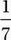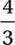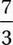# SAT Math Multiple Choice Question 717: Answer and Explanation

### Test Information

Question: 717

12. 3x2 = 4x + c

In the equation above, c is a constant. If x = -1 is a solution of this equation, what other value of x satisfies the equation?

• A.• B.• C.• D. 7

Explanation:

C

We can find the value of c by just substituting x = -1 into the equation.

Given equation:

3x2 = 4x + c

Substitute x = -1:

3(-1)2 = 4(-1) + c

Simplify:

3 = -4 + c

7 = c

Therefore, the equation is:

3x2 = 4x + 7

Subtract 4x and 7:

3x2 - 4x - 7 = 0

Factor using Sum-Product Method:

(x + 1)(3x - 7) = 0

(Notice that the factor (x + 1) corresponds to the fact that x = -1 is a solution to the quadratic.)

Use Zero Product Property to find other solution:

3x - 7 = 0